### tileset 2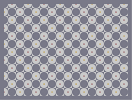Hover over the thumbnail for a full-size version.

Author Slightly_Thwumped_up author:slightly_thwumped_up tileset unrated 2007-05-13 3 more votes required for a rating. \$tileset 2#Slightly_Thwumped_up#none#=87:=87:=87:=87:=87:=876;<96;<96;<96;<96;<96;<7:=87:=87:=87:=87:=87:=<96;<96;<96;<96;<96;<96=87:=87:=87:=87:=87:=876;<96;<96;<96;<96;<96;<7:=87:=87:=87:=87:=87:=<96;<96;<96;<96;<96;<96=87:=87:=87:=87:=87:=876;<96;<96;<96;<96;<96;<7:=87:=87:=87:=87:=87:=<96;<96;<96;<96;<96;<96=87:=87:=87:=87:=87:=876;<96;<96;<96;<96;<96;<7:=87:=87:=87:=87:=87:=<96;<96;<96;<96;<96;<96=87:=87:=87:=87:=87:=876;<96;<96;<96;<96;<96;<7:=87:=87:=87:=87:=87:=<96;<96;<96;<96;<96;<96=87:=87:=87:=87:=87:=876;<96;<96;<96;<96;<96;<7:=87:=87:=87:=87:=87:=<96;<96;<96;<96;<96;<96=87:=87:=87:=87:=87:=876;<96;<96;<96;<96;<96;<7:=87:=87:=87:=87:=87:=<96;<96;<96;<96;<96;<96=87:=87:=87:=87:=87:=876;<96;<96;<96;<96;<96;<7:=87:=87:=87:=87:=87:=|0^24,72!0^72,120!0^120,168!0^168,216!0^216,264!0^264,312!0^312,360!0^360,408!0^408,456!0^456,504!0^504,552!0^312,552!0^264,504!0^216,456!0^168,408!0^120,360!0^72,312!0^24,264!0^24,456!0^72,504!0^120,552!0^168,24!0^216,72!0^264,120!0^312,168!0^360,216!0^408,264!0^456,312!0^504,360!0^552,408!0^600,456!0^648,504!0^696,552!0^744,408!0^696,360!0^648,312!0^600,264!0^552,216!0^504,168!0^456,120!0^408,72!0^360,24!0^552,24!0^600,72!0^648,120!0^696,168!0^744,216!0^744,24!12^24,552!12^24,360!12^72,408!12^120,456!12^168,504!12^216,552!12^24,168!12^72,216!12^120,264!12^168,312!12^216,360!12^264,408!12^312,456!12^360,504!12^408,552!12^600,552!12^552,504!12^504,456!12^456,408!12^408,360!12^360,312!12^312,264!12^264,216!12^216,168!12^168,120!12^120,72!12^72,24!12^264,24!12^312,72!12^360,120!12^408,168!12^456,216!12^504,264!12^552,312!12^600,360!12^648,408!12^696,456!12^744,504!12^744,312!12^696,264!12^648,216!12^600,168!12^552,120!12^504,72!12^456,24!12^648,24!12^696,72!12^744,120# the second in my tilset maps... heres the link to the first one http://numa.notdot.net/map/88866 oh and after much thought i kept it a tile set

## Other maps by this author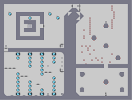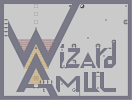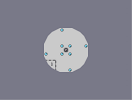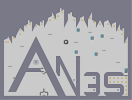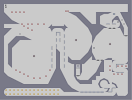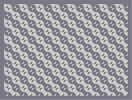where the cross roads end Wizard_Amul survival ring AN3S gersand fernad tileset 1